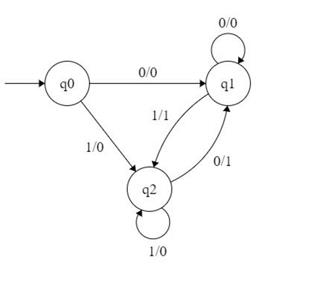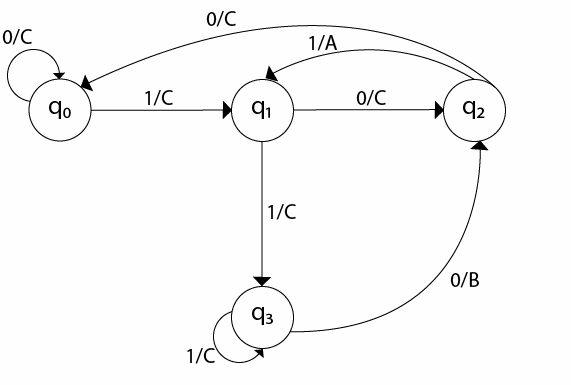# What is a mealy machine in TOC?

In a mealy machine, the output symbol depends upon the present input symbol and on the present state of the machine.

The output is represented with each input symbol and each state is separated by /.

The mealy machine can be described by 6 tuples

(Q, q0, Σ, O, δ, λ')

Where,

• Q: Finite set of states
• q0: Initial state of machine
• Σ: Finite set of input alphabet
• O: Output alphabet
• δ: Transition function, where Q × Σ → Q
• λ': Output function, where Q × Σ →O

The length of output for a mealy machine is equal to the length of input.

## Example 1

Input − 11

Transition −  δ (q0,11)=> δ(q2,1)=>q2

Output − 00 (q0 to q2 transition has Output 0 and q2 to q2 transition also has Output 0)

The transition diagram is as follows −## Explanation

• Step 1 − q0 is the start state on input ‘0’, it goes to state b and it generates an output ‘0’ and on input ‘1’ it goes to state q2 and generates an output ‘0’.
• Step 2 − q1 on input ‘0’ goes to q1 itself generating output ‘0’ and on ‘1’ goes to q2 generates an output ‘1’.
• Step 3 − q2 on input ‘1’ goes to q2 itself and generates an output ‘0’ and on ‘0’ goes to state q1 generating output ‘1’.

The state table of a Mealy Machine is shown below −

Present stateNext State
Input =0Input =1
StateOutputStateOutput
->q0q10q20
q1q10q21
q2q11q20

## Example 2

Design a Mealy machine for a binary input sequence {0,1}

If the input has a substring 101, the output is A.

If the input has substring 110, its output is B Otherwise, its output is C.

The transition diagram is as follows −The state’s transition table is as follows −

Present stateNext State
Input =0Input =1
StateOutputStateOutput
->q0q0Cq1C
q1q2Cq3C
q2q0CAC
q3q2Bq3C